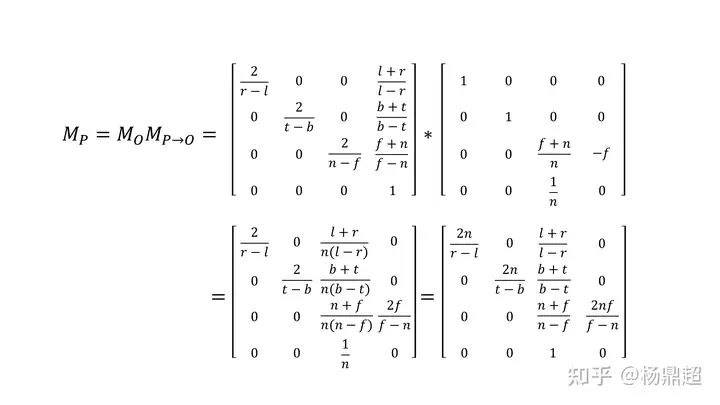0%

# 空间变换的意义

• 描述摄像机运动
• 描述模型运动

## 视图变换

• 3D到2D的投影变换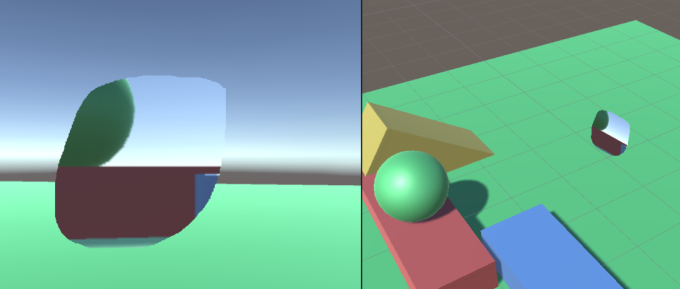# 二维变换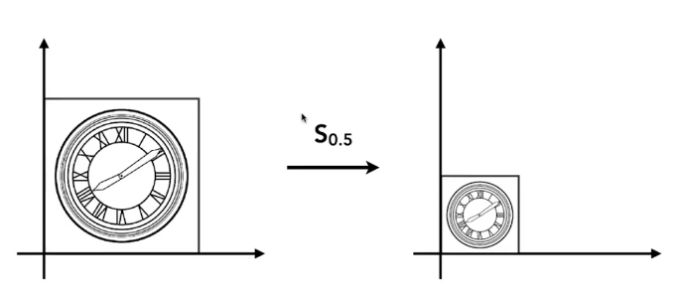x`=sx

y`=sy

• y轴反转：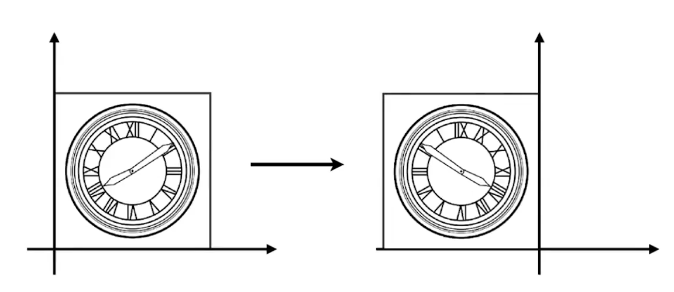x`=-x

y`=y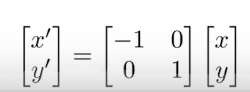• 斜变化：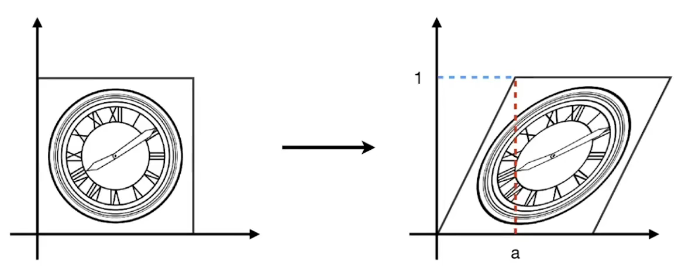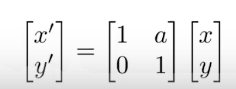• 旋转变化：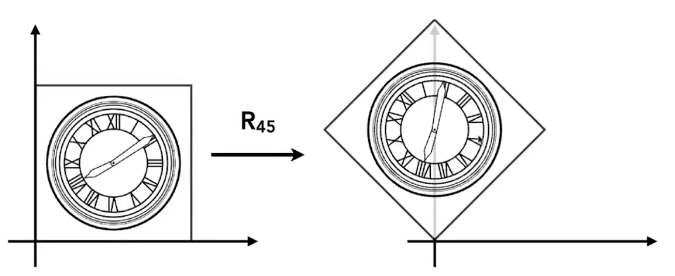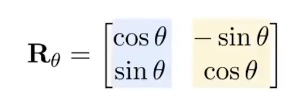# 齐次坐标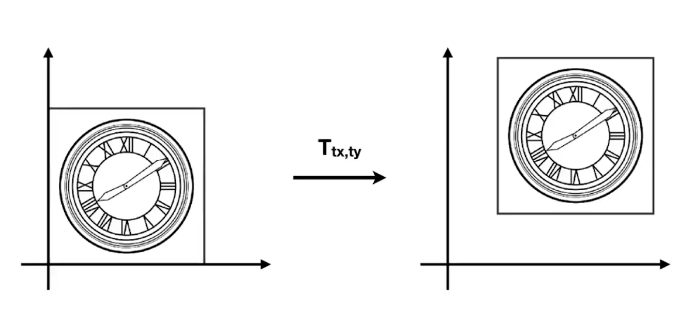• 2D point =(x,y,1)T
• 2D vector =(x,y,0)T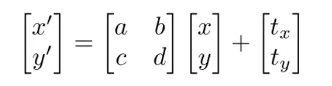• vector + vector = vector
• point - point = vector
• point + vector = point
• point + point = ??

• 逆变换：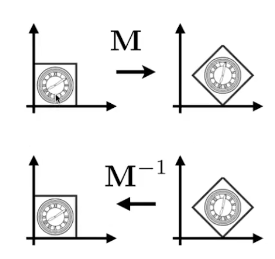# 组合变换

1. 复杂的变换通过简单变换组合得来
2. 简单变换的顺序很重要（很形象：矩阵乘法不满足交换律）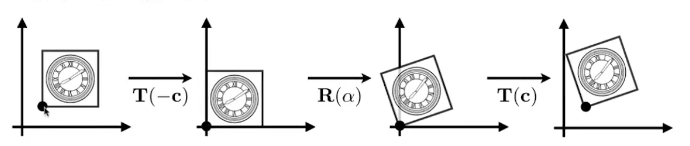# 三维变换

• 3D point =(x,y,z,1)T
• 3D vector =(x,y,z,0)T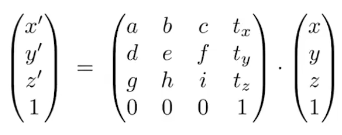• 绕轴旋转变换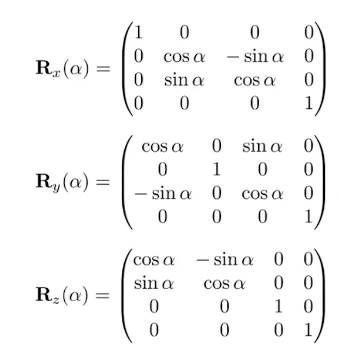• 普遍旋转

Rxyz(a,b,c) = Rx(a)Ry(b)Rz(c)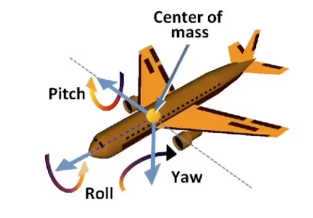//

# 观测变换

View/Camera Transformation

# 投影变换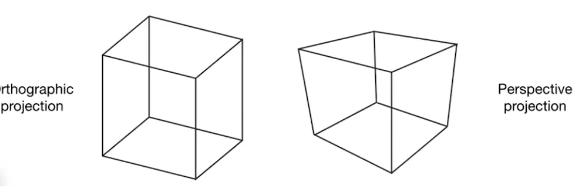## 正交

• 将相机摆放到原点，布置好方向，朝向等
• 去除z轴（会出现无法区分物体前后的情况）
• 按比例移动并拉扯成到[-1,1]2的矩形上（转换为规范视域体，为了方便处理，无需考虑屏幕尺寸）

• 用AABB包围盒（6个参数）转换为规范视规体

OpenGL为(-1,-1,-1)到(1,1,1)

DirectX为(-1,-1,0)到(1,1,1)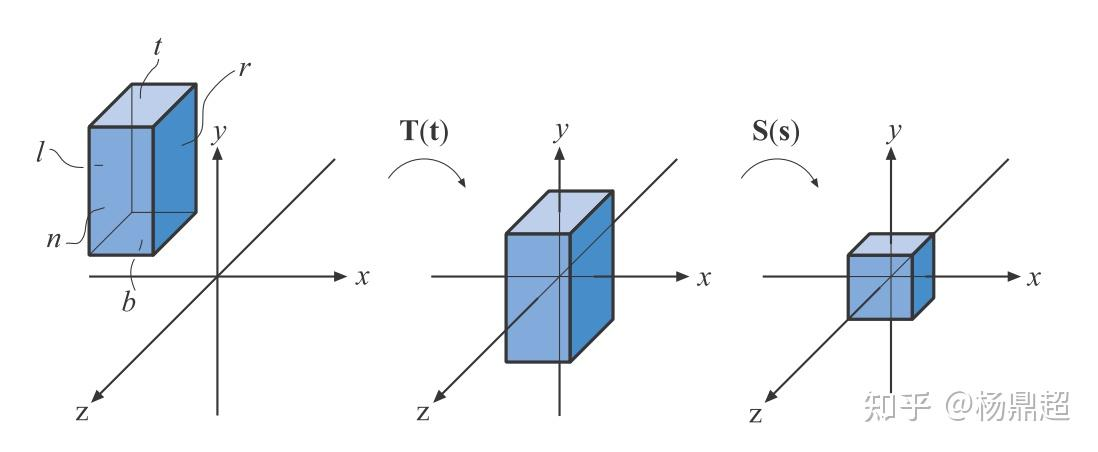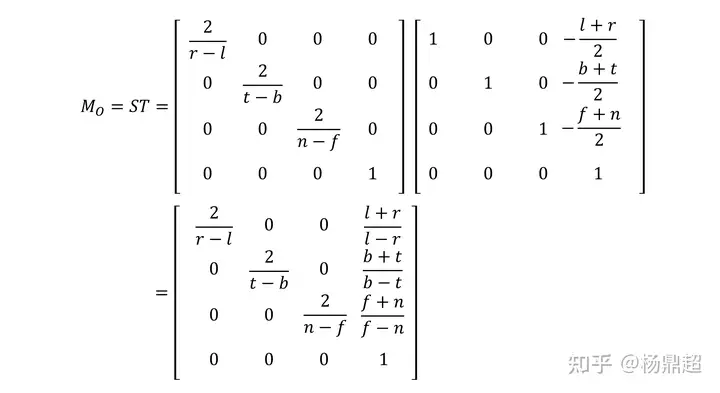## 透视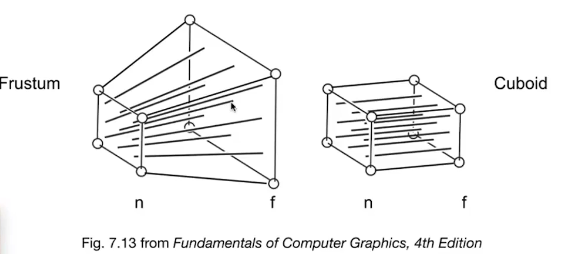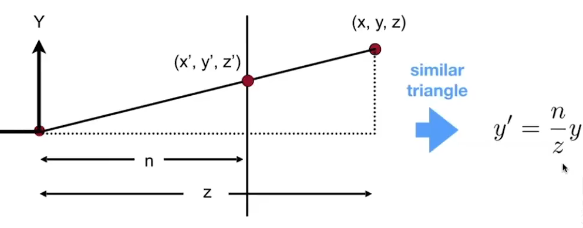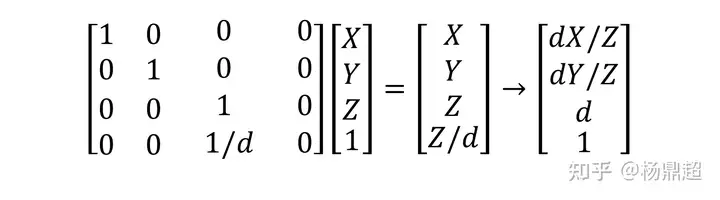发现问题：Z不见了，发生了和正交一样的情况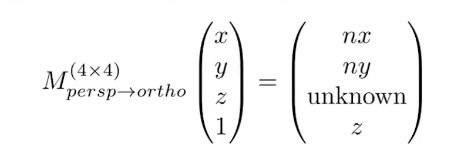• 统计所有常规点位变换规律（就是上图）
• 统计近裁面上的所有点（也就是(x,y,z,1)T=W(x,y,z,1)T)
• 统计z轴上的点，z轴值不变